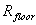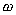Appendix A - Design Calculations

i)                    Torus

Floor area required at 1g: 1,442,640m2

Floor area of torus:Where= radius to torus floor

Rotation rate required to get 1g acceleration at torus floor:Where= acceleration due to gravity (9.806ms­-2)= angular velocity (rad/sec)= radius to torus floor

Limit of rotation rate: 1 rpm = 0.1047 rad/sec

=> minimum radius to torus floor: 894.5m

Line of sight (i.e. - how far you can see before floor reaches eye level)

Equation of a circle: x2 + y2 = r2

Where x and y are co-ordinates of point, i.e. - ordered pair (x, y) and r = radius of circle

For line of sight, x = radius - height above floor (1.7m),  r = radius to torus floor

=> Line of sight (y) =Minor radius (incl. shielding) 100m 125m 150m Radius to torus floor (m) 1183.52 940.997 780.96 Line of sight (m) 63.412 56.54 51.5 rpm required for 1g 0.869 0.9748 1.07

ii)                   Moveable spheres (see fig. 7.1b)

Floor area required: 9,000m2  (2,250m2 per sphere)

Door to lift: every 18m

Area of sleeves:= 86.59m2 in each floor

Floor 2 (door at centre) => floor 1.5m below equator.

x2 + y2 = r2

=>        x2 = r 2 - (1.5)2

=> Floor area =Floor 1, 16.5m above equator

Floor area =Floor 3, 19.5m below equator

Floor area =Floor area required = S floor areas 1, 2, 3

=>2250 ==> r 2    =  484.545

r       =  22m